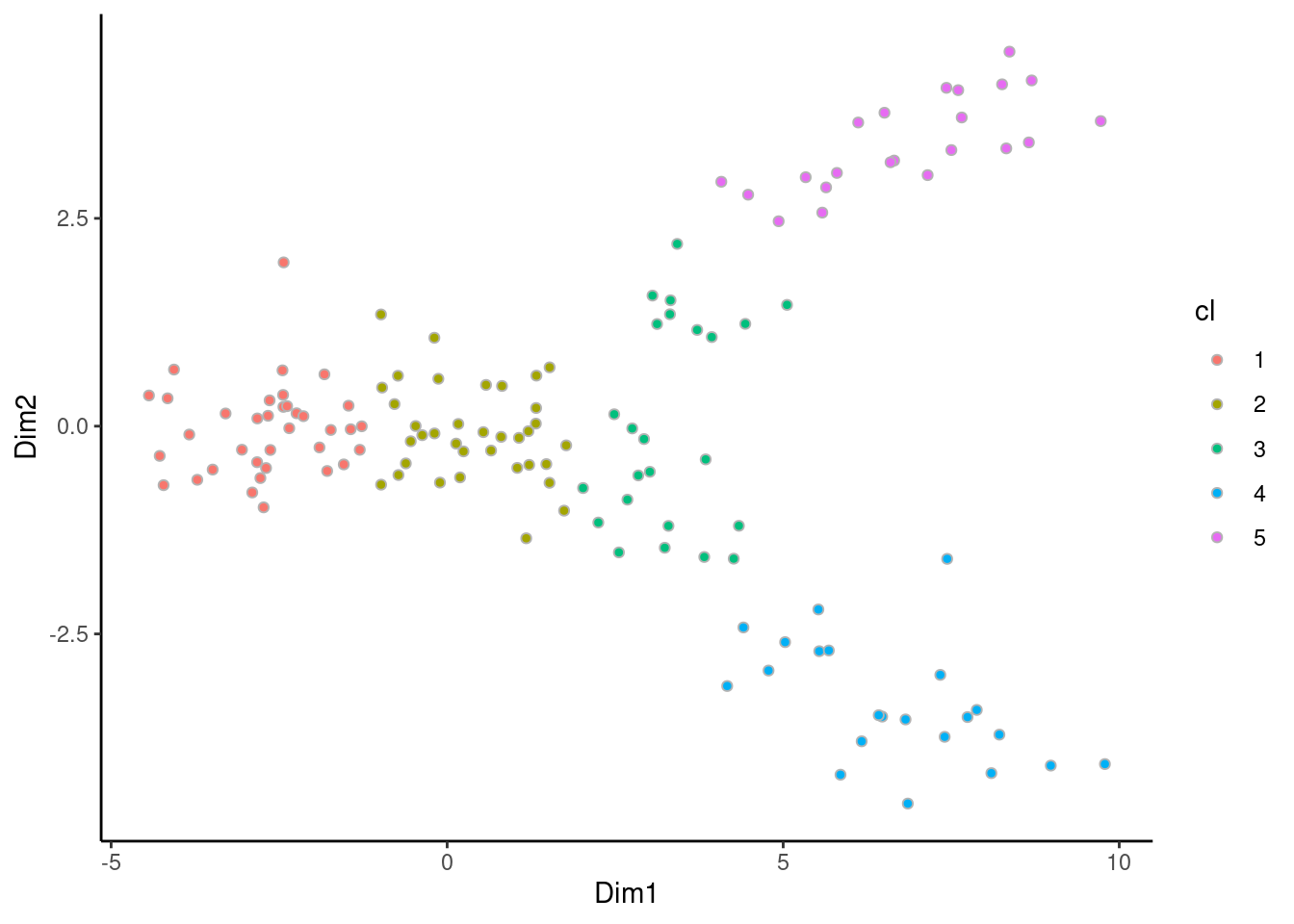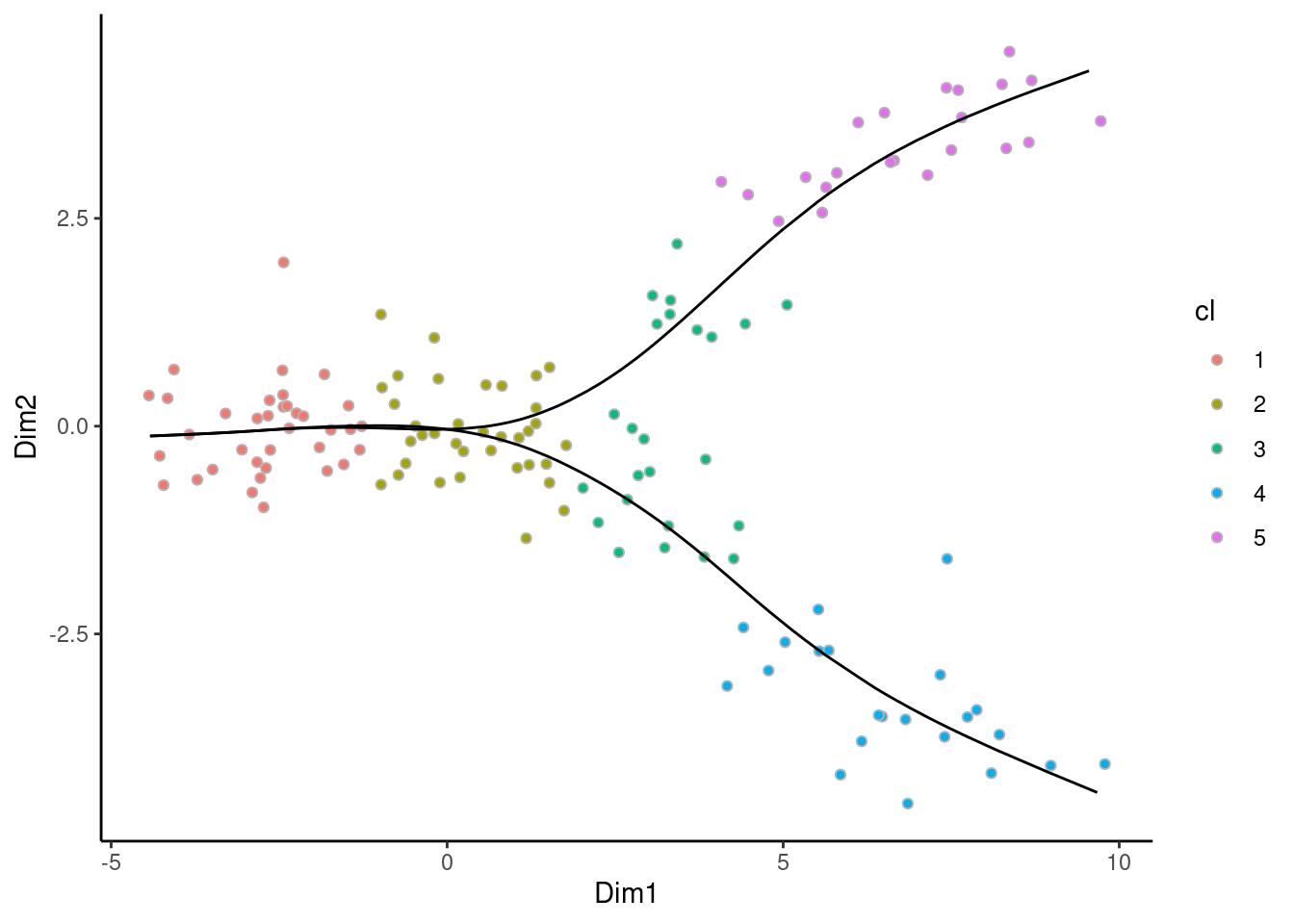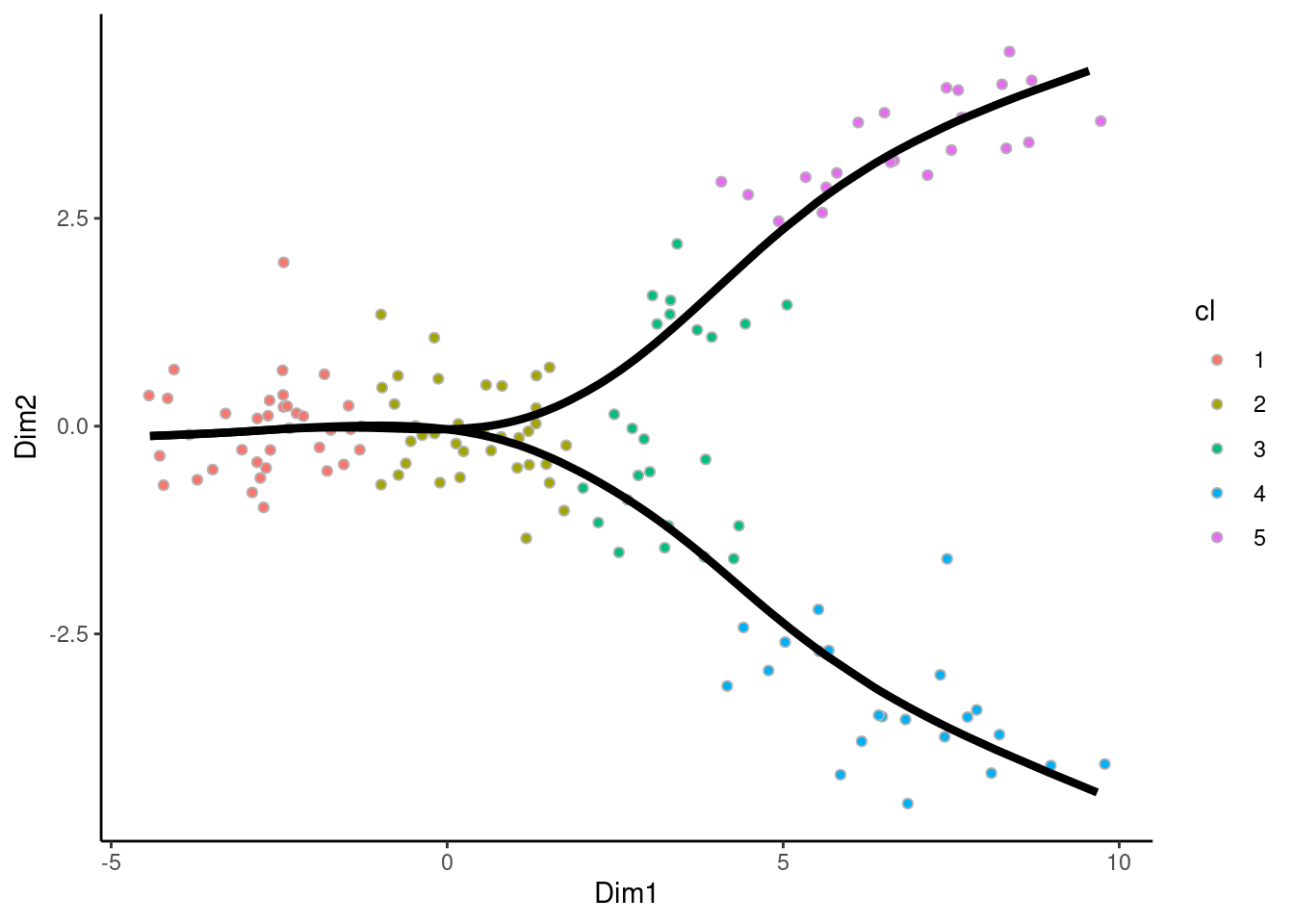# Introduction

`Slingshot`(Street et al. 2018) is a popular and powerful trajectory inference tool, available from bioconductor. While it only provides default plotting functions in base R, the package also offer conversion functions that can be used to generate plots with `ggplot2`(Wickham 2016).

We will assume that you are both familiar with `slingshot` and `ggplot2`. Otherwise, please refere to the respective packages’ vignettes.

# Running slingshots

We run `slingshot` on the provided example

``````# Running slinghsot
suppressPackageStartupMessages(library(slingshot))
data("slingshotExample")
rd <- slingshotExample\$rd
colnames(rd) <- c("Dim1", "Dim2")
cl <- slingshotExample\$cl
df <- data.frame(rd, "cl" = as.character(cl))
sds <- slingshot(rd, cl)``````

We can then visualize the samples in reduced dimension.

``````suppressPackageStartupMessages(library(ggplot2))
suppressPackageStartupMessages(library(dplyr))
# Plotting the results
p <- ggplot(df, aes(x = Dim1, y = Dim2)) +
geom_point(aes(fill = cl), col = "grey70", shape = 21) +
theme_classic()
p``````# Adding the curves to a ggplot2 object

Then, we can add the curves. We can extract the needed information using the accessory functions. This can be turned into tidy data.frames that interact nicely with `ggplot2` by setting the `as.df` argument to `TRUE`.

``````curves <- slingCurves(sds, as.df = TRUE)
p + geom_path(data = curves %>% arrange(Order),
aes(group = Lineage)) ``````We can do all the usual ggplot2 operations

``````p + geom_path(data = curves %>% arrange(Order),
aes(group = Lineage), size = 1.5) ````````````p + geom_path(data = curves %>% arrange(Order),
aes(group = Lineage, col = as.character(Lineage)), size = 1.5) ``````